# Bolt Online Learning Toolbox¶

Release: 1.4 October 13, 2010 peter.prettenhofer@gmail.com

Contents:

Introduction

Bolt features discriminative learning of linear predictors (e.g. SVM or Logistic Regression) using fast online learning algorithms. Bolt is aimed at large-scale, high-dimensional and sparse machine-learning problems. In particular, problems encountered in information retrieval and natural language processing.

Bolt considers linear models (bolt.model.LinearModel) for binary classification,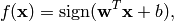and generalized linear models (bolt.model.GeneralizedLinearModel) for multi-class classification,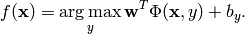Where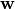and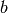are the model parameters that are learned from training data. In Bolt the model parameters are learned by minimizing the regularized training error given by,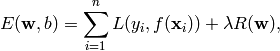where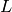is a loss function that measures model fit and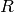is a regularization term that measures model complexity.

Features

Bolt supports the following trainers for binary classification:

• Supports various loss functions: Hinge, Modified Huber, Log.
• Supports various regularization terms: L2, L1, and Elastic Net.
• PEGASOS (bolt.trainer.sgd.PEGASOS)

For multi-class classification:

Benchmark

The following RCV1-CCAT benchmark results show that Bolt is competitive to state-of-the-art linear SVM solvers such as SVMPerf, liblinear, or sgd. The dataset comprises 781.264 training documents, each represented by a 47.152 dimensional feature vector.

Algorithm Training time Accuracy
SVMlight >600.00 sec
SVMPerf  11.60 sec 94.79
liblinear  9.00 sec 94.77
bolt  2.33 sec 94.79
sgd  1.09 sec 94.77
  Uses C=1000
  Uses SVM (Dual), B=1
  Uses E=5, r=0.00001, l=0, b
  Uses epochs=5, lambda=0.00001